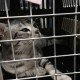# 浅谈Heatmap

## 捕捉点击

```<script>

\$(document).mousedown(function(e) {
if (e.clientX >= \$(window).width() || e.clientY >= \$(window).height()) {
return;
}

\$.get("/path/to/a/empty/html/file", {
page_x       : e.pageX,
page_y       : e.pageY,
screen_width : screen.width,
screen_height: screen.height
});
});
});

</script>```

```<script>

var image = new Image();
image.src = "...";

</script>```

## 分析日志

`page_x=...&page_y=...&screen_width=...&screen_height=...`

```#!/usr/bin/awk -f

BEGIN {
FS="&";
}

NF == 4 {
param["page_x"]        = "0";
param["page_y"]        = "0";
param["screen_width"]  = "0";
param["screen_height"] = "0";

split(\$0, query, "&");

for (key in query) {
split(query[key], item, "=");
if (item in param) {
param[item] = item;
}
}

print "page_x:"       , param["page_x"];
print "page_y:"       , param["page_y"];
print "screen_width:" , param["screen_width"];
print "screen_height:", param["screen_height"];

print "\n";
}```

## 渲染图片

```<?php

\$coordinates = array();

for (\$i = 0; \$i < 1000; \$i++) {
\$coordinates[] = array(rand(\$i, 1000), rand(\$i, 1000));
}

\$max_repeat = max(
array_count_values(
array_map(function(\$v) { return "{\$v}x{\$v}"; }, \$coordinates)
)
);

\$opacity = 1 - 1 / \$max_repeat;

\$heatmap_image = new Imagick();

\$heatmap_image->newImage(1000, 1000, new ImagickPixel('white'));
\$heatmap_image->setImageFormat('png');

\$plot_image = new Imagick('plot.png');

\$iterator = \$plot_image->getPixelIterator();
foreach(\$iterator as \$row) {
foreach (\$row as \$pixel) {
\$colors = \$pixel->getColor();
foreach (array('r', 'g', 'b') as \$channel) {
\$color = \$colors[\$channel];
if (\$color !== 255) {
\$colors[\$channel] = \$color + ((255 - \$color) * \$opacity);
}
}

\$pixel->setColor("rgb({\$colors['r']},{\$colors['g']},{\$colors['b']})");
}

\$iterator->syncIterator();
}

\$plot_size = \$plot_image->getImageGeometry();

foreach (\$coordinates as \$pair) {
\$heatmap_image->compositeImage(
\$plot_image,
Imagick::COMPOSITE_MULTIPLY,
\$pair - \$plot_size['width'] / 2,
\$pair - \$plot_size['height'] / 2
);
}

\$color_image = new Imagick('clut.png');

\$heatmap_image->clutImage(\$color_image);

\$heatmap_image->writeImage('heatmap.png');

?>```

## 最终展示

BTW：热点可能会随着时间改变，为了能对照某个时间的网页，可以使用CutyCapt截屏。顺手再贴一个相关的项目：smt2（simple mouse tracking）。

## 《浅谈Heatmap》上有22个想法

1.KnightE说道：

虽说是“浅谈”，不过至少打开了一扇门。有机会在线上业务采样试试。

2. Pingback引用通告： Tweets that mention 浅谈Heatmap | 火丁笔记 -- Topsy.com

3.神仙说道：

每点一下，请求一次也太夸张了点。

•老王说道：

恩，神仙说得有理。还可以更简单点，只做个频率限制，比如每秒钟仅允许一次点击，免得有些患有网游综合症的人，喜欢不停的点击鼠标。

4. Pingback引用通告： 网站点击热图的技术实现 | 标点符

5. Pingback引用通告： 网站点击热图的技术实现 - Your Need is Me

6.Lc说道：

8.soar说道：
10.aisensiy说道：